# Bayesian Coin Flip¶

## Introduction¶

This notebook gives an introduction to Bayesian statistics, using mostly elementary probability theory and mathematics.

In :
from sympy import *
from sympy.stats import *


## Coin Flipping¶

Imagine we have a coin $c$ with two sides (heads $\mathrm{H}$ and tails $\mathrm{T}$). The coin may be fair, or it may biased, we don't know. We will say that $p(c=\mathrm{H})$ is the probability of the coin toss facing heads, and $p(c=\mathrm{T}) = 1 - p(c=\mathrm{H})$ is the probability of tails. The value of $h := p(c=\mathrm{H})$ itself is uncertain, and can take any value from 0 to 1. We represent this uncertainty by a probability density function $f(h)$. $f$ is a distribution of distributions: The value of $f(h)$ determines the bias of the coin, which itself determines the distribution of the coin tosses. The following image illustrates this distribution of distributions for a factory of fair coins. Any particular coin may have a bias (although coins with a large bias are rare), but most coins are fair within a margin of error.

In :
h = Symbol("h")
f = Beta(h, 10, 10)
fig = plot(density(f)(h), (h, 0, 1), title="Probability density function of the probability of heads", xlabel="$h$", ylabel="$f(h)$")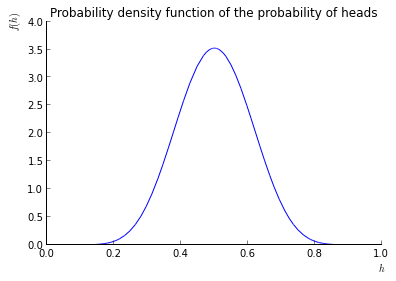After sampling a specific coin from $f(h)$, we can then represent the coin toss with a discrete random variable.

In :
c = Symbol("c")
bias = sample(f)
C = Coin(c, bias)
print density(C)
print [sample(C) for i in range(10)]

{H: 0.511884213785308, T: 0.488115786214692}
[H, T, T, T, H, H, H, T, T, H]


## Bayesian Inference¶

Our goal is to understand the consequences of observing tosses of a coin with unknown bias $\hat{h}$. Ultimately, we want to know the bias of the coin itself, but to answer that, we look at a much bigger question: What is the probability density function $f(h)$ of a coin factory that produces coins with this bias? Of course, any factory that assigns a non-zero probability mass to $\hat{h}$ is a possible answer, but as we will see in the next section, some are more reasonable than others. Let's first assume that by some kind of trick we came up with a guess of such a prior distribution $f_n$ for our factory. Then the Bayesian inference process allows us to improve this guess, based on an observed coin toss $c_n$ (heads or tails). The resulting posterior distribution $f_{n+1}$ is the best estimate for the probability density function of our hypothetical factory, given the prior distribution and the observed data. It is derived by Bayes' theorem for conditional propabilities:

$$f_{n+1}(\hat{h}) := f_n(h=\hat{h}|c=c_n) = \frac{p(c=c_n|h=\hat{h}) f_n(h=\hat{h})}{p(c=c_n)}, \hat{h}\in [0, 1]$$

The left hand side is the posterior distribution after one Bayesian update at step $n$ (there are as many steps as there are observed data points). It represents the updated probability of the coin bias being $\hat{h}$ after observing $c_n$. The parameter $\hat{h}$ varies over the whole range $[0,1]$, giving us a probability mass for each possible bias of the coin. On the right hand side, we have the likelihood $p(c=c_n|h=\hat{h})$ of the observed data $c_n$, assuming that the bias of the coin is $\hat{h}$, and the prior distribution for $h$. To get a proper probability density function, we have to make sure that the right hand side sums to $1$ over all possible values for $\hat{h}$. For this, we divide by the normalizing constant, which is easily expressed by the sum over all possible numerators:

$$p(c=c_n) = \int_0^1 p(c=c_n|h) f_n(h) \thinspace dh$$

Don't panic. We will do these calculations several times for specific values of $f_n$ and $c_n$, which will give you plenty of time to review how the Bayesian update process works and what its effects are on the probability density function of the coin bias.

## Prior Distribution¶

To get started with Bayesian inference, we need a prior distribution of the probability function of our coin factory. If we had complete knowledge of the bias of the coin, this would be a simple Dirac delta function:

In :
f_exact = DiracDelta(h-bias)
# Note: DiracDelta is not a Sympy distribution, so we can not plot the density function or sample it.
integrate(f_exact, (h, 0, 1))

Out:
1

If we lack any information, we can assume that the coin comes from a uniform distribution:

In :
f_uniform = Uniform(h, 0, 1)
sample(f_uniform)
# This craps out on me.
# plot(density(f_uniform)(h), (h, 0, 1))

Out:
0.583601108813623

Choosing the uniform distribution is not as random as it may appear. The uniform distribution has maximum entropy. In this sense it ensures that the prior does not include any hidden assumptions that are not justified by the model. However, sometimes it can be useful to choose other priors, and in general it may be a good idea to verify that the inference process is not overly sensitive to different reasonable choices for the prior. We will revisit this question at the very end.

## Step 1: The Uniform Prior¶

Because we assume no knowledge about the bias of the coin in question, we use a uniform prior:

$$f_1(h) := 1$$
In :
f1 = 1


## Step 2: The First Coin Toss¶

We toss the coin and get heads:

$$c_1 = H$$

We now want to perform the first Bayesian update. For this, let's look at the individual components of Bayes Theorem. Clearly, the prior probability of any bias $\hat{h}$ is $1$, because of the chosen uniform distribution:

$$f_1(\hat{h}) = 1 \text{ (uniform prior)}$$

The likelihood of observing heads, given the bias $\hat{h}$, is simply $\hat{h}$, because this is how we defined the bias. Note that the likelihood of tails would be $1-\hat{h}$. These are the only two possible outcomes for the likelihood in this example.

$$p(c=H|h=\hat{h}) = \hat{h} \text{ (by definition)}$$

Now we only need to calculate the normalization constant. Luckily, we already did most of the work above:

$$\int_0^1 p(c=H|h) \cdot f_1(h) \thinspace dh = \int_0^1 h \cdot 1 \thinspace dh = \left. \frac{1}{2} h^2 \right |_0^1 = \frac{1}{2}$$

Putting it all together, we get the posterior distribution:

$$f_2(\hat{h}) := f_1(h=\hat{h}|c=H) = \frac{\hat{h} \cdot 1}{\frac{1}{2}} = 2\hat{h}$$

or simply: $f_2(h) = 2h$

In :
f2 = h * f1 / integrate(h * f1, (h, 0, 1))
plot(f2, (h, 0, 1), title="Posterior distribution after observing $c=(\mathrm{H})$.", xlabel="$h$", ylabel="$f_2(h)$")
f2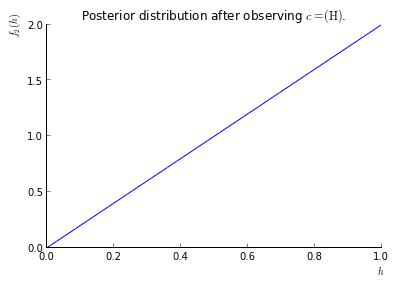Out:
2*h

We can see that the posterior distribution favors heads-biased coins over tails-biased coins, in stark contrast to the uniform distribution. However, even extremely tails-biased coins are still possible, with one exception: Coins that exclusively show tails are now excluded ($f_2(0) = 0$). This makes sense, because we already observed heads once.

### Tails¶

Because of the symmetry of the problem, we would get the same result if we had seen tails, but flipped horizontally (due to the transformation $p(c=\mathrm{T}) = 1 - h$).

In :
plot(f2.subs(h, 1-h), (h, 0, 1), title="Posterior distribution after observing $c=(\mathrm{T})$.", xlabel="$h$", ylabel="$f_2(h)$")
f2.subs(h,1-h)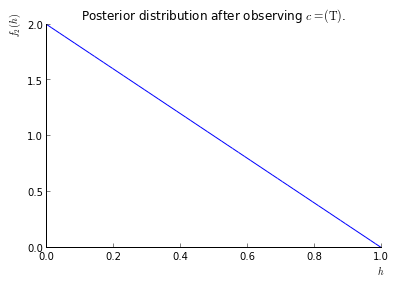Out:
-2*h + 2

## Step 3: The Second Coin Toss¶

We toss the coin a second time, and we get heads again. Now we repeat the process. The previous posterior distribution now becomes the new prior, which requires recalculating the normalization constant. However, the likelihood is the same as in the previous step.

$$\int_0^1 p(c=H|h) \cdot f_2(h) \thinspace dh = \int_0^1 h \cdot 2\hat{h} \thinspace dh = \left. \frac{2}{3} h^3 \right |_0^1 = \frac{2}{3}$$

Which yields:

$$f_3(\hat{h}) := f_2(h=\hat{h}|c=H) = \frac{\hat{h} \cdot 2\hat{h}}{\frac{2}{3}} = 3\hat{h}^2$$

or simply: $f_3(h) = 3h^2$

In :
f3 = h * f2 / integrate(h * f2, (h, 0, 1))
fig = plot(f3, (h, 0, 1), title="Posterior distribution after observing $c=(\mathrm{HH})$.", xlabel="$h$", ylabel="$f_3(h)$")
f3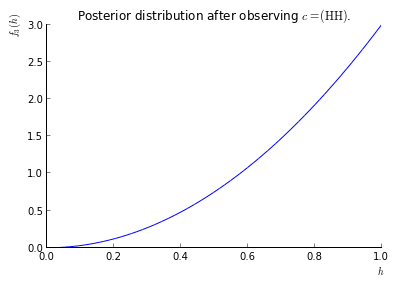Out:
3*h**2

We can see that the distribution now favors heads-biased coins even more strongly. Considering that two heads in a row is a common event even for fair coins, this may seem strange. But recall that we used a uniform prior at the very beginning, and did not favor fair coins over strongly biased coins at all. Consequently, the Bayesian inference process choses the most favorable distribution based on the uniform prior and the observed data. Had we chosen a prior that preferred fair coins over biased ones (incorporating external knowledge about the world, coin tossing, and gravity), we would see a more moderate result here. We will see in the folowing sections that, as more data comes in, the choice of the prior becomes less dominant on the overall result.

### ... or Tails¶

Had we observed tails in the second toss, we would get a very different distribution. The integrals are easy to solve, so we will just give the result here as computed with Sympy. Now that tails is observed, the distribution misses the strong leaning towards heads-biased coins, as it must vanish at $h=1$ (i.e. all-heads).

In :
f3_ = (1-h) * f2 / integrate((1-h) * f2, (h, 0, 1))
plot(f3_, (h, 0, 1), title="Posterior distribution after observing $c=(\mathrm{HT})$.", xlabel="$h$", ylabel="$f_3(h)$")
f3_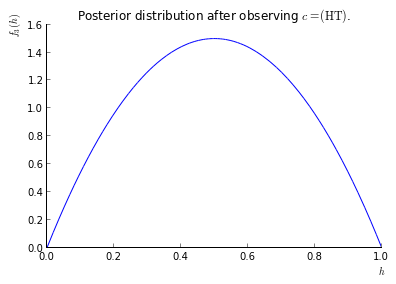Out:
6*h*(-h + 1)

## Step 4: The Third Coin Toss¶

We give the results for three coin tosses (apart from identities and symmetries):

In :
f4 = h * f3 / integrate(h * f3, (h, 0, 1))
fig = plot(f4, (h, 0, 1), title="Posterior distribution after observing $c=(\mathrm{HHH})$.", xlabel="$h$", ylabel="$f_4(h)$")
f4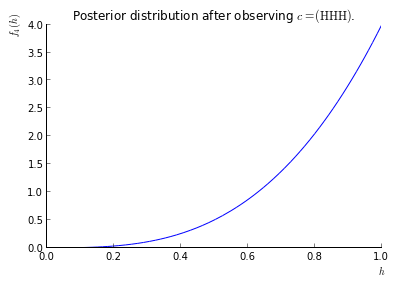Out:
4*h**3
In :
f4_ = (1-h) * f3 / integrate((1-h) * f3, (h, 0, 1))
fig = plot(f4_, (h, 0, 1), title="Posterior distribution after observing $c=(\mathrm{HHT})$.", xlabel="$h$", ylabel="$f_4(h)$")
f4_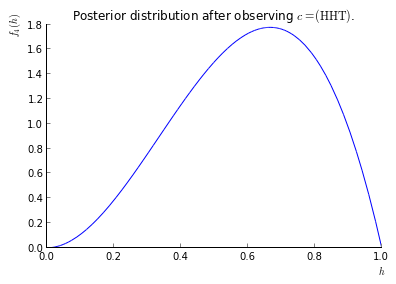Out:
12*h**2*(-h + 1)

## Arbitrary Number of Coin Tosses¶

The final posterior distribution of a Bayesian update process does not depend on the order of the updates, or even if we do the updates incrementally one after another, or all at once. We can use this to calculate the posterior after any number of coin tosses $c_1, \..., c_n$. Assume that the number of heads is $H$ and the number of tails is $T$, then, choosing the uniform prior and any order of the data, we have:

$$f_1(\hat{h}) = 1$$$$p(c_{1,\...,n}|h=\hat{h}) = h^H (1-h)^T$$$$\int_0^1 h^H (1-h)^T \cdot 1 \thinspace dh = \text{ ?}$$

Unfortunately, we now have to calculate the integral, which is a bit tricky using only elementary methods (try it, if you are up for a challenge!). Luckily, Sympy can spit out an acceptable answer in a couple of minutes. Note that we simplify the expression, and also move the expression to the denominator, where it will end up anyway:

In :
H = Symbol("H", positive=True, integer=True)
T = Symbol("T", positive=True, integer=True)
# Warning, slow!
#expr = integrate(h**H*(1-h)**T, (h, 0, 1))
expr = gamma(H + 1)*hyper((-T, H + 1), (H + 2,), exp_polar(2*I*pi))/gamma(H + 2)
beta = expr.simplify()
beta

Out:
gamma(H + 1)*gamma(T + 1)/gamma(H + T + 2)

So, we end up with:

$$\int_0^1 h^H (1-h)^T \cdot 1 \thinspace dh = \frac{1}{B(H+1,T+1)}$$

with

$$B(p, q) := \frac{\Gamma(p) \Gamma(q)}{\Gamma(p+q)}$$

and consequently:

$$f(h) = \frac{1}{B(H+1,T+1)}h^H(1-h)^T$$

Please say hello to the Beta-Distribution!

Let's look at this function for a couple of examples:

In :
f = (1/beta) * h**H * (1-h)**T
fig = plot(f.subs({H:2,T:1}), (h, 0, 1), title="Posterior distribution after observing $c=(\mathrm{HHT})$.", xlabel="$h$", ylabel="$f(h)$")
fig = plot(f.subs({H:10,T:1}), (h, 0, 1), title="Posterior distribution after observing $c=(H=10,T)$.", xlabel="$h$", ylabel="$f(h)$")
fig = plot(f.subs({H:10,T:10}), (h, 0, 1), title="Posterior distribution after observing $c=(H=10,T=10)$.", xlabel="$h$", ylabel="$f(h)$")
fig = plot(f.subs({H:100,T:50}), (h, 0, 1), title="Posterior distribution after observing $c=(H=100,T=50)$.", xlabel="$h$", ylabel="$f(h)$")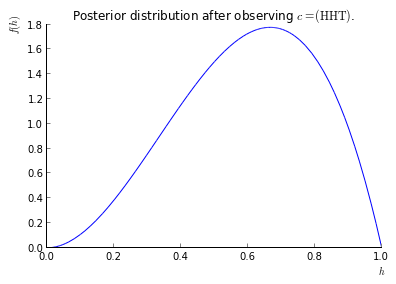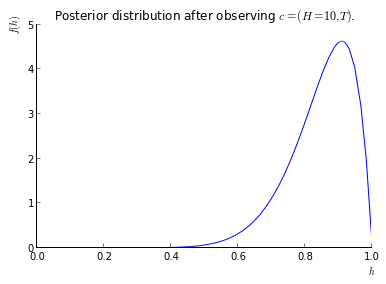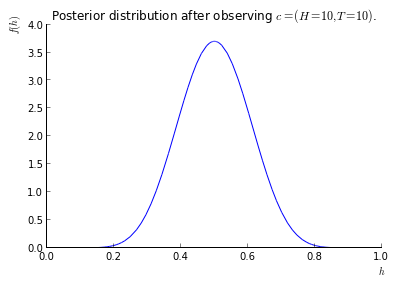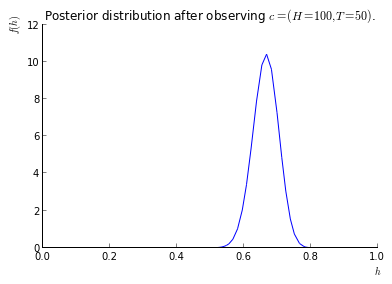## Prior Distribution, revisited¶

To illustrate what can go wrong if an unreasonable prior distribution is chosen, imagine we were to use the Dirac delta function $f(h) = \delta(h_0 - h)$ as prior distribution. In this case, the integral in the denominator would collaps to $p(c|h=\hat{h})$, which would cancel out with the likelihood, leaving the prior distribution unchanged in the posterior. We can understand this intuitively: The delta function represents an absolute prejudice in the value of $h$, and this belief is so strong that no factual data can change it. In this sense, the Bayesian inference process works as expected. To avoid such a situation, the prior should give at least some probability mass to any reasonable outcome, and let the data speak for itself. We could see above that after a coin toss facing heads, the posterior vanishes at $0$, which means that the Bayesian inference process excluded the possibility for an all-tails coin. Likewise, after a coin toss facing tails, all-heads coins are excluded. No harm was done by including those possibilities in the prior, and the inference process quickly adapted to the data and excluded impossible choices.

## What just happened¶

We saw that starting with a uniform prior, the Baysian inference process can give us a lot of information about the certainty of a random variable, even after just a couple of observations. The result can be improved incrementally when new data arrives, or in batches. For a coin flip, the result is a Beta distribution, where the parameters indicate the number of heads and tails in the data. The choice of the prior is significant, but as more data arrives, it fades into the background. A strong bias in the prior that is not justified by the model may be hard to overcome.

A limitation of the process is that it assumes i.i.d. (independent and identically distributed) random variables. If there was some correlation in the data, or the bias of the coin changes over time, we would prefer to look at some other method (for example Markov Chain).

Bayesian inference is one of the foundations of machine learning, where it has many applications, for example testing emails for spam.

In [ ]: